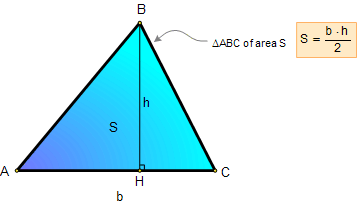Gergonne's TheoremGergonne's Theorem: Triangle and Concurrent Cevians. Level: High School, SAT Prep, College
 In the figure below, given a triangle ABC, AD, BE, and CF are concurrent cevians at G. Prove that:FACTS AND HINTS:
Geometry theorem proving is one of the most challenging skills for students to learn. When a proof requires auxiliary construction, the difficulty of the problem increases drastically, perhaps because deciding which construction to make is an ill-structured problem. By “construction,” we mean adding geometric figures (points, lines, planes) to a problem figure that wasn’t mentioned as "given."

1. CEVIAN: A cevian is a line segment which joins a vertex of a triangle with a point on the opposite side (or its extension).

2. AREA OF A TRIANGLE:

Proposition: The area of a triangle equals one-half the product of the length of a side and the length of the altitude to that side..
 Home | Geometry | Search | Problems Email | By Antonio Gutierrez Last updated: July 17, 2009